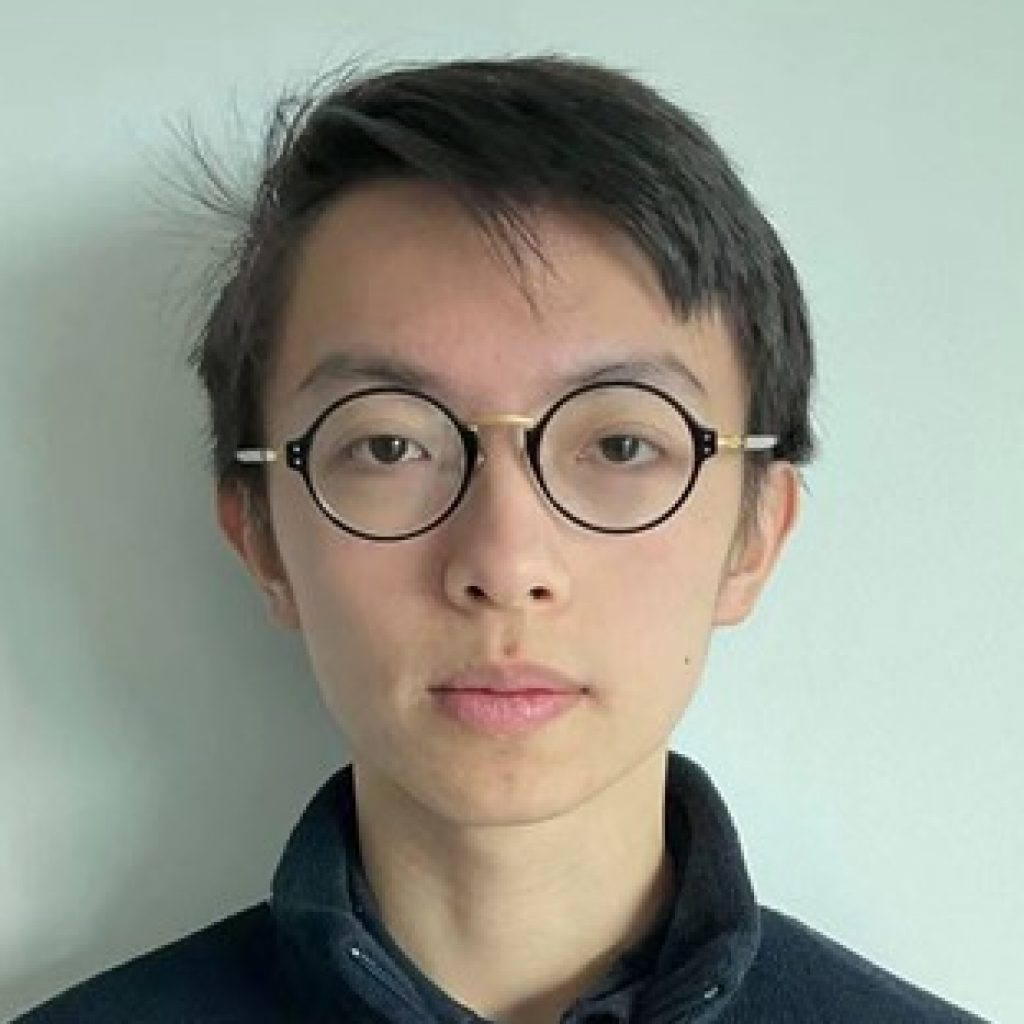We explore a basic topological characteristic associated with supersymmetric theories, called the Witten index. The project starts with recalling basic concepts of classical mechanics including Lagrangian and action. Then this is extended to quantum mechanics, in which we construct a supersymmetrised version of a specific model.

From there we explore some characteristic properties of supersymmetric theories including conserved charges, the Hamiltonian, and a Z2 grading. Then the concepts of supersymmetric ground states and supersymmetry breaking are intergraded, which naturally leads to the definition of the Witten index.

Around the 1970s, people discovered a new type of symmetry in quantum field theory which establishes a relationship between bosons and fermions. This is called supersymmetry. Several experiments have been conducted in places like Large Hadrom Collider (LHC) in order to answer questions in this field, like “Is supersymmetry, an extension of the Standard Model, realized in nature, implying that all known particles have supersymmetric partners?” In my project, we try to understand the mathematical fundament of this question using a toy model of a supersymmetric theory. Firstly, we explore a special Lagrangian that is invariant under supersymmetry. Then we analyze several properties like conserved charges and Hamiltonian.

After that, we move from the classical to the quantum system. We promote the Hamiltonian and the conserved charges to operators on a Hilbert space. We can characterise the Hilbert space in terms of energy levels given by the eigenvalues of the Hamiltonian. From there we explore the additional structure of the supersymmetric Hilbert space and introduce the concept of the Witten index which was first introduced by Edward Witten. Here the idea that every particle has a supersymmetric super partner at higher energy levels will appear, and that’s what people are aiming to observe using LHC.

To compute the Witten index we explore how to derive an expression for the ground state of a supersymmetric theory. Also, we apply our methods to an example called a supersymmetric harmonic oscillator in three ways to show that it actually works and the results are consistent.

There are several possibilities for further research. We can further explore the mathematical notion of a Morse function that comes up in this context, and the concepts can also be applied to further, more complicated models such as the supersymmetric version of a multi-particle system on theories in higher dimensions.

Yixiang Wang
The University of MelbourneView Yixiang Wang
Profile